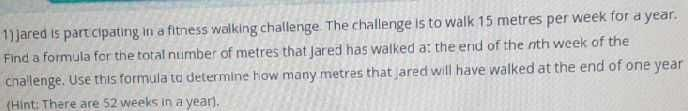### ¿Todavía tienes preguntas de matemáticas?

Pregunte a nuestros tutores expertos
Algebra
Pregunta1] jared is part cipating in a fitness walking challenge. The challenge is to walk $$15$$ metres per week for a year. Find a formula for the total number of metres that Jared has walked a: the erid of the nth week of the cnallenge. Use this formula td determine how many metres that ared will have walked at the end of one year (Hint: There are $$52$$ weeks in a year).

$$d= 15n\\n= 52\Rightarrow d= 780$$